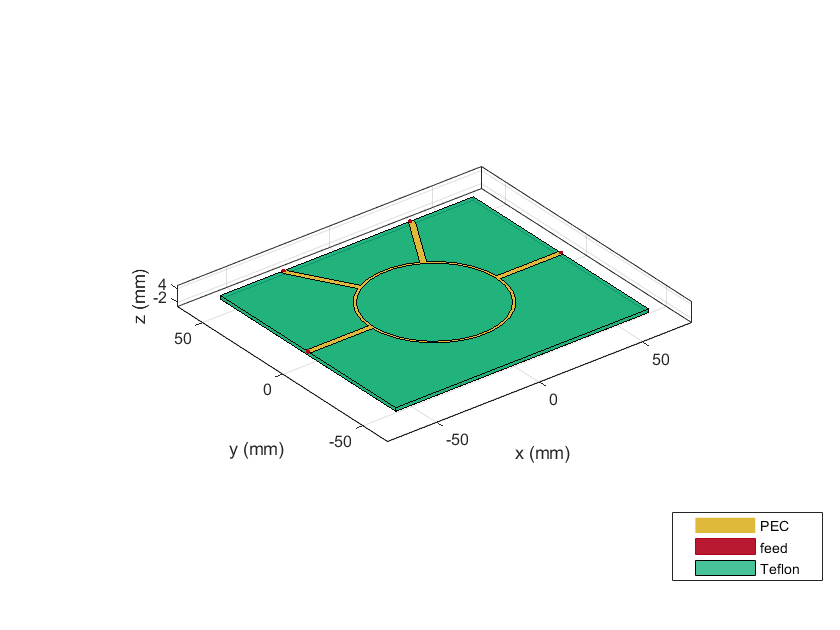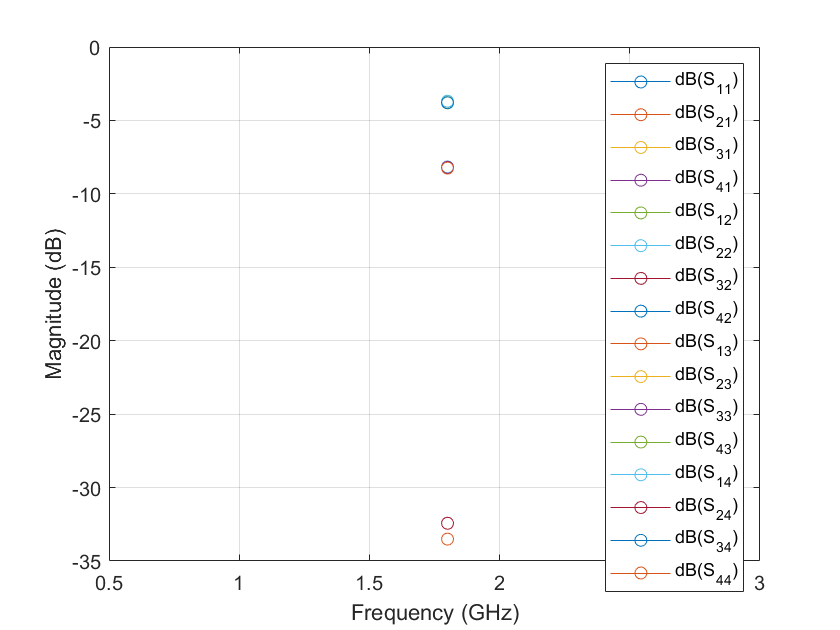# design

Design rat-race coupler around specified frequency

Since R2021b

## Syntax

``coupler = design(couplerobj,frequency)``
``coupler = design(___,Name=Value)``

## Description

````coupler = design(couplerobj,frequency)` designs a rat-race coupler around the specified frequency.```

example

````coupler = design(___,Name=Value)` designs a rat-race coupler line with additional options specified using name-value arguments. NotePCB components designed using the `design` function operate around the specified frequency with a 10-15% tolerance. ```

## Examples

collapse all

Design a rat-race coupler at 1.8 GHz and with a charecteristic impedance of 75 ohms.

```coupler = design(couplerRatrace,1.8e9,Z0=75); show(coupler);```Plot the S-parameters of the coupler at 1.8 GHz.

```spar = sparameters(coupler,1.8e9); rfplot(spar)```## Input Arguments

collapse all

Rat-race coupler, specified as a `couplerRatrace` object.

Example: `coupler = couplerRatrace; design(coupler,2e9)` designs a rat-race coupler around a frequency of 2 GHz.

Design frequency of the rat-race coupler, specified as a real positive scalar in hertz.

Example: `2.5e9`

Data Types: `double`

### Name-Value Arguments

Specify optional pairs of arguments as `Name1=Value1,...,NameN=ValueN`, where `Name` is the argument name and `Value` is the corresponding value. Name-value arguments must appear after other arguments, but the order of the pairs does not matter.

Example: `Z0=70`

Characteristic impedance of the coupler in ohms, specified as a positive scalar.

Data Types: `double`

## Output Arguments

collapse all

Rat-race coupler operating around the specified reference frequency, returned as a `couplerRatrace` object.

## Version History

Introduced in R2021b